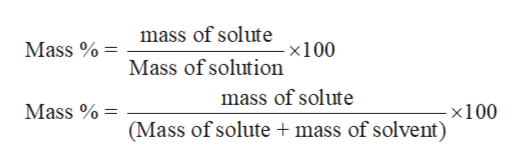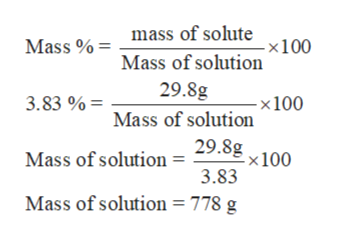# Determine the mass of 3.83% AgNO3 solution that contains 29.8 g of AgNO3_____g solution

Question

Determine the mass of 3.83% AgNO3 solution that contains 29.8 g of AgNO3

_____g solution

check_circleExpert Solution
Step 1

Molality is the way to express the concentration of any solution. It can be defined as the number of moles of solute dissolves in 1 kg of the solvent. The mathematical expression for molality can be written as:

Step 2

Mass percent or weight percent of a solution is the mass of the solute by the mass of the solution multiply by 100. The mathematical expression for molality can be written as:help_outlineImage Transcriptionclosemass of solute x100 Mass % Mass of solution mass of solute Mass % -x100 (Mass of solute mass of solvent) fullscreen
Step 3

Calculate the mass ...help_outlineImage Transcriptionclosemass of solute Mass %= - x100 Mass of solution 29.8g 3.83 %= - x 100 Mass of solution 29.8g -x 100 Mass of solution = 3.83 Mass of solution = 778 g fullscreen

### Want to see the full answer?

See Solution

#### Want to see this answer and more?

Solutions are written by subject experts who are available 24/7. Questions are typically answered within 1 hour*

See Solution
*Response times may vary by subject and question
Tagged in

### Other